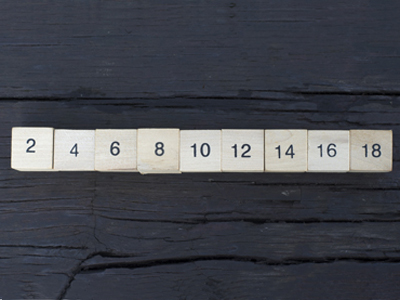These are the simplest kind of Number Series.

# VR - Number Series Using Arithmetic and Geometric Progression

So far we have looked at two forms which Number Series can take: Alternating Terms and Alternating Terms with Geometric Progression. Now we come to some much simpler types: ones which use arithmetic, and those which use geometric progression but without alternating.

If you have already read the previous two articles, then you’ll find this one much less taxing. For anybody with even the barest maths skills, these forms of Number Series should be a doddle.

As always, let’s show you exactly what I mean with some examples.

Example Question One

Use the following series of numbers to determine what should come next.

 3 6 9 12 (?)

In these sorts of papers, you are, again, going to receive possible answers and are expected to choose from a selection of five. In some school entry systems this may vary but encourage a child who does not see the answer to work out which answers are not likely to be accurate and try to use the possible answers to guide thinking. For these particular questions I shall simply explain the technique rather than suggest five answers.

In the example the connection between the first and second digits could be one of two things:

It could be a case of ‘double the first number to make the second’, or…

It could be ‘add three to make the next number’.

Using the other numbers, we can see that it must be the latter as each number increases by three so the answer will be 15.

This is known as an arithmetic progression. It uses addition or subtraction to get to the next figure. The other main type of progression involves multiplying and that is known as a geometric progression. Most examples you will find in verbal reasoning papers will be variations of arithmetic progressions.

Now let’s look at geometric progression:

Example Question Two

Use the following series of numbers to determine what should come next.

 2 4 8 16 (?)

This time you must stop your child from assuming that the link between each number is going to be based on addition. Adding two to the first digit will get you to the second, but then not to the third. However, multiplying each number by two will get you to the next number. The next number in this series will be double 16 – so 32.

This can be presented in different ways but the prime one to look out for is the 'powers of two' series which is what's in the example. It never hurts to have your child aware of the next few numbers in the sequence - 32, 64, 128, 256, 512 and 1024. Other variations can include the use of 3 or another number as a starting point, doubling throughout, e.g.

Use the following series of numbers to determine what should come next.

 3 6 12 24 (answer 48)

...or multiplying by a different number, not just 2. However, the numbers get very high very quickly so there are only a few possible questions that are likely to be presented in this style. For example:

Use the following series of numbers to determine what should come next.

 2 6 18 54 (answer 162)

In this sequence the multiplier is 3 as each number is three times the preceding one so the answer would be 54 x 3 = 162.

## Sample Tests

Now that you’ve learned so much about Number Series in the Eleven Plus Verbal Reasoning exam, your child should have no trouble answering these kinds of question. If you’d like to find out, then direct them to the four quizzes we have on the topic. Here are some links:

Number Series 1

Number Series 2

Number Series 3

Number Series 4

There is one more style of Number Series - negative arithmetic progression – which we will look at in the next exam illustration. In the meantime, good luck!

We use cookies to make your experience of our website better.

To comply with the new e-Privacy directive, we need to ask for your consent -